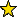# PseudomonomialPrimaryDecomposition -- primary decomposition of a square free pseudomonomial ideal

## Description

A pseudomonomial is a polynomial in K[x1,x2,...,xn] that can be written as a product of factors of the form (xi-ai)^ni, where ai is 0 or 1. The xi's in the product should be distinct. A square free pseudomonomial ideal is an ideal generated by pseudomonomials such that each ni=1.

This package finds the primary decomposition of square free pseudomonomial ideals. It also determines if an ideal is a pseudomonomial ideal.

For example, x1^2*(x3-1) is a pseudomonomial, but not square free. The polynomial x1*(x3-1) is a square free pseudomonomial. The ideal ideal(x1*(x3-1),(x1-1)*(x2-1)*x4,x1*x2*x3,(x1-1)*x2*(x5-1)) is a square free pseudomonomial ideal.

 i1 : R = ZZ/2[x1,x2,x3,x4,x5]; i2 : I = ideal(x1*(x3-1),(x1-1)*(x2-1)*x4,x1*x2*x3,(x1-1)*x2*(x5-1)); o2 : Ideal of R i3 : isSquarefreePseudomonomialIdeal I o3 = true i4 : C = primaryDecompositionPseudomonomial I o4 = {ideal (x5 + 1, x1, x2 + 1), ideal (x2, x3 + 1, x1 + 1), ideal (x1, x4, ------------------------------------------------------------------------ x2), ideal (x1, x4, x5 + 1), ideal (x3 + 1, x4, x2)} o4 : List i5 : intersect C == I o5 = true

## CertificationVersion 0.3 of this package was accepted for publication in volume 12 of The Journal of Software for Algebra and Geometry on 18 July 2022, in the article Primary decomposition of squarefree pseudomonomial ideals. That version can be obtained from the journal or from the Macaulay2 source code repository.

## Version

This documentation describes version 0.3 of PseudomonomialPrimaryDecomposition.

## Source code

The source code from which this documentation is derived is in the file PseudomonomialPrimaryDecomposition.m2.

## For the programmer

The object PseudomonomialPrimaryDecomposition is .